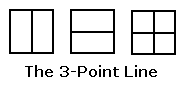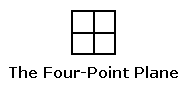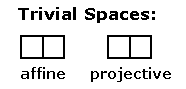Finite Geometry Notes   | Home | Site Map | Author |

Geometry Simplified

by Steven H. Cullinane, June 24, 2010(a projective space)

The above finite projective space is the simplest nontrivial example
of a Galois geometry (i.e., a finite geometry with coordinates in a
finite (that is, Galois) field.)

The vertical (Euclidean) line represents a (Galois) point, as does the horizontal line
and also the vertical-and-horizontal cross that represents the first two points'
binary sum (i.e., symmetric difference, if the lines are regarded as sets).

Homogeneous coordinates for the points of this line —

(1,0), (0,1), (1,1).

Here 0 and 1 stand for the elements of the two-element Galois field GF(2).

The 3-point line is the projective space corresponding to the affine space
(a plane, not a line) with four points —(an affine space)

The (Galois) points of this affine plane are not the single and combined (Euclidean)
line segments that play the role of points in the 3-point projective line,
but rather the four subsquares that the line segments separate.

For further details, see Galois Geometry.

There are, of course, also the trivial two-point affine space
and the corresponding trivial one-point projective space —Here again, the points of the affine space are represented by squares,
and the point of the projective space is represented by a line segment
separating the affine-space squares.